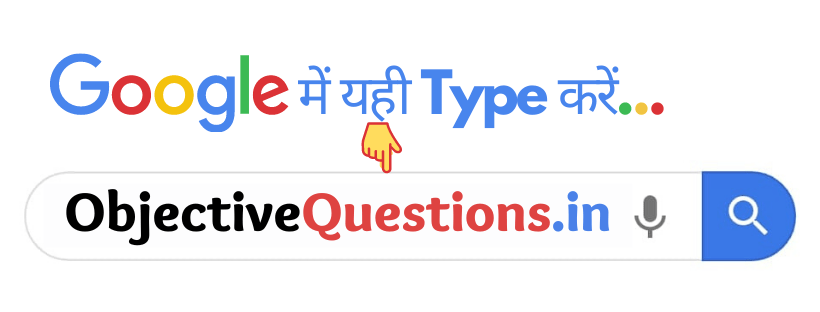# CHAPTER-6: WORK, ENERGY, AND POWER

1. The commercial unit of energy is-

• Watt
• Joule
• Kilowatt-hour
• None of these
Kilowatt-hour

2. The product of force and displacement is called –

• Energy
• Momentum
• Power
• Work
Work

3. Work will be said not to be done if the force and displacement are –

• parallel
• acting in the opposite direction
• perpendicular
• None of these
acting in the opposite direction

4. Energy is-

• the capacity for doing work
• rate of doing work
• change of work
• None of these
the capacity for doing work

5. Potential energy of an object of mass ‘m’ at a height ‘h’ is –

• 1/2mv²
• m²gh
• mgh
• mg
mgh

6. The expression of kinetic energy is –

• 1/2mv²
• m²gh
• mgh
• mg
mgh

7. Out of the following, which is not the unit of energy –

• Joule
• Calorie
• Kilo-Watt
• Electron-Volt
Kilo-Watt

8. Joule is a unit of –

• Work and Power
• Power and Energy
• Force
• Work and Energy.
Work and Energy.

9. Area under the Force-Displacement curve represents –

• Velocity
• Impulse
• Acceleration
• Work done
Work done

10. The momentum of a body increased by 25%, then kinetic energy is increased by about –

• 25 %
• 56 %
• 5 %
• 38 %
56 %

11. The rate of doing work is called –

• energy
• force
• power
• pressure
power

12. What will be the kinetic energy of a substance when its velocity is doubled?

• Double
• Four times
• Half
• One-fourth
Four times

13. What will be the kinetic energy of a body when its velocity is halved?

• Double
• Four times
• Half
• One-fourth
One-fourth

14. Watt is the unit of the following –

• Work
• Power
• Energy
• None of these